# 電路學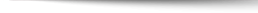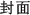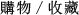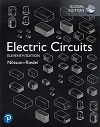## Electric Circuits 11/e

+作者：

### Nilsson

+年份：
2020 年11 版
+ISBN：
9781292261041
+書號：
EE0465PC
+規格：

+頁數：
816
+出版商：
Pearson(Asia)

\$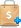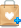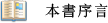The fundamental goals of the best-selling Electric Circuits remain unchanged. The 11th Edition continues to motivate students to build new ideas based on concepts previously presented, to develop problem-solving skills that rely on a solid conceptual foundation, and to introduce realistic engineering experiences that challenge students to develop the insights of a practicing engineer. The 11th Edition represents the most extensive revision since the 5th Edition with every sentence, paragraph, subsection, and chapter examined and oftentimes rewritten to improve clarity, readability, and pedagogy–without sacrificing the breadth and depth of coverage that Electric Circuits is known for. Dr. Susan Riedel draws on her classroom experience to introduce the Analysis Methods feature, which gives students a step-by-step problem-solving approach.●Practical Perspectives introduce chapters and provide real-world circuit examples. Every chapter begins by describing a practical application of the chapter content. At the end of each chapter, the Practical Perspective is revisited by performing a quantitative circuit analysis using the newly introduced material.
Originating from author Susan Riedel’s own classroom experience, Analysis Methods are ‘recipes’ for students to help them get started on a problem with step-by-step directions that guide them to a problem’s solution.
Chapter Problems reinforce problem solving as fundamental to the study of circuit analysis. Approximately 30% of the problems in the 11th edition are new or were rewritten.
Assessment Problems prompt students at key points in the chapter, asking them to stop and assess their mastery of a particular objective by solving one or more assessment problems.
Fundamental Equations and Concepts are set apart from the main text to help readers focus on the key principles and help navigate through the important topics.
Examples in every chapter illustrate the concepts presented in the text in the form of a numeric example. There are nearly 200 examples in this text, an increase of about 35% when compared to the previous edition. The examples illustrate the application of a particular concept and often employ an Analysis Method, and exemplify good problem-solving skills.
Integration of Computer Tools assists students in the learning process by providing a visual representation of a circuit’s behaviour, validating a calculated solution, reducing the computational burden of more complex circuits, and iterating toward a desired solution using parameter variation. Chapter problems suited for exploration with PSpice and Multisim are marked accordingly.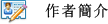James Nilsson - Professor Emeritus Iowa State University
Susan Riedel - Marquette University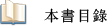1. Circuit Variables
2. Circuit Elements
3. Simple Resistive Circuits
4. Techniques of Circuit Analysis
5. The Operational Amplifier
6. Inductance, Capacitance, and Mutual Inductance
7. Response of First-Order RL and RC Circuits
8. Natural and Step Responses of RLC Circuits
9. Sinusoidal Steady-State Analysis
10. Sinusoidal Steady-State Power Calculations
11. Balanced Three-Phase Circuits
12. Introduction to the Laplace Transform
13. The Laplace Transform in Circuit Analysis
14. Introduction to Frequency Selective Circuits
15. Active Filter Circuits
16. Fourier Series
17. The Fourier Transform
18. Two-Port Circuits
Appendix A:  The Solution of Linear Simultaneous Equations
Appendix B:  Complex Numbers
Appendix C:  More on Magnetically Coupled Coils and Ideal Transformers
Appendix D:  The Decibel
Appendix E:  Bode Diagrams
Appendix F:  An Abbreviated Table of Trigonometric Identities
Appendix G:  An Abbreviated Table of Integrals
Appendix H:  Common Standard Component Values